Next: Three-Dimensional Airfoils Up: Incompressible Aerodynamics Previous: Vortex Sheets

# Induced Flow

A vortex filament is necessarily associated with fluid flow circulating about the filament. Let us determine the relationship between the filament vorticity and the flow field that it induces. This problem is mathematically identical to determining the magnetic field generated by a current filament. In the latter case, the Maxwell equation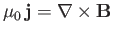(9.57)

can be inverted to give the well-known Biot-Savart law (Fitzpatrick 2008)(9.58)

Here,is the current density, andthe magnetic field-strength. By analogy, given that vorticity is related to fluid velocity via the familiar relation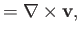(9.59)

we can write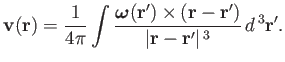(9.60)

This expression allows us to determine the flow field induced by a given vorticity distribution. In particular, for a vortex filament of intensity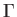the previous expression reduces to(9.61)

where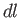is an element of length along the filament. Likewise, for a vortex sheet of intensity per unit length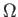, we obtain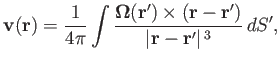(9.62)

where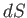is an element of area of the sheet.Next: Three-Dimensional Airfoils Up: Incompressible Aerodynamics Previous: Vortex Sheets
Richard Fitzpatrick 2016-03-31